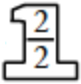### Home > CCAA > Chapter 4 Unit 3 > Lesson CC2: 4.2.3 > Problem4-53

4-53.

Simplify the following expressions.

1. $\frac{2}{5}-\frac{1}{6}$

You must find a common denominator before you can combine these terms.

A common denominator is often a multiple of both denominators of the fractions that are to be combined.
A good one to use for this expression would be $30$.

1. $\frac{3}{7}-\frac{7}{14}$

$\frac{3}{7}+$$\frac{6}{14}$

$\frac{6}{14}-\frac{7}{14}=-\frac{1}{14}$

$-\frac{1}{14}$

1. $\frac{5}{8}-\frac{2}{3}$

Refer to part (a).